2

# 優化梯度下降

## Momentum

`Momentum`顧名思義主要運用動量移動到最底端，例如下圖丟一顆紅球，地心引力往下帶動紅球，到底部時累積的速度可讓紅球往上爬，這時候就有機會擺脫不是全域最佳解狀態，如果使用`SGD`則到底部時則不再往上爬，這時候可能會導致不是全域最佳解狀態。而這方法稱為`指數加權衰減量`(依地心引力原理就是計算重力+速度)。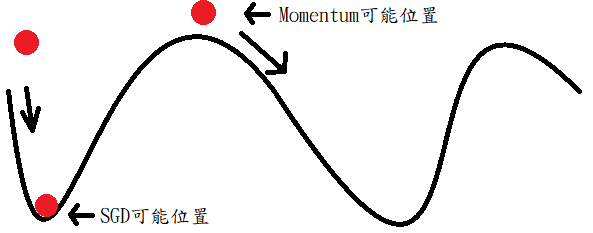### 公式

使用不同動量與相對的學習率畫出學習的震盪。這裡參考的點子實作了一次如下圖，可以看到m=0.9時震盪就比m=0好很多，但這裡你應該也會發現這四種的m和學習率相加等於1而1這部分實際上我們並不知道是多少，也就是說可以選擇m=0.9..0.8而總和是N，學習率就是(N-m)，所以到目前為止學習率還是要去猜測的。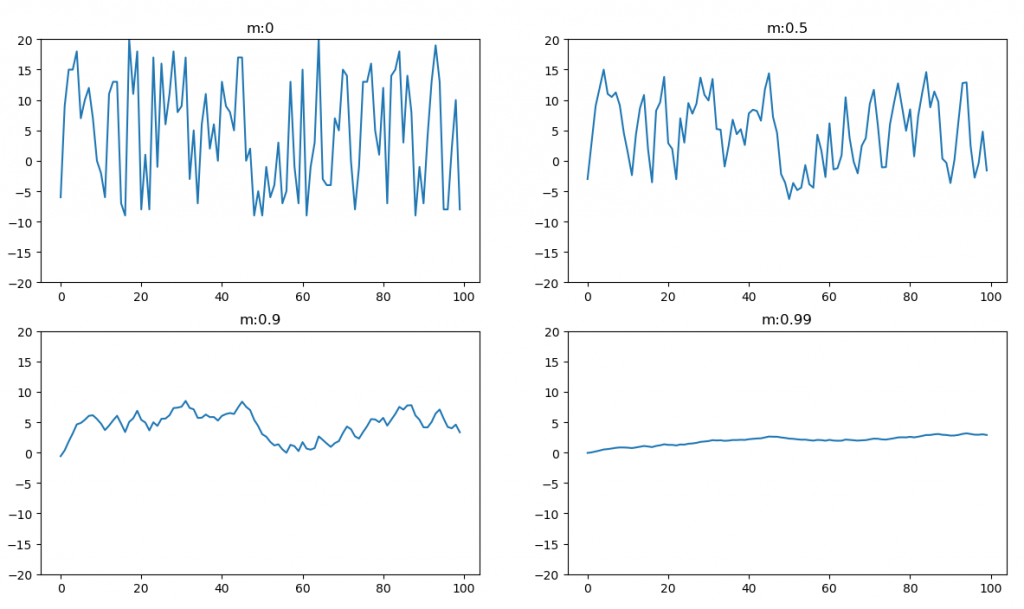``````momentum = [0, 0.5, 0.9, 0.99]
stand = np.random.randint(-20, 10, 100)

for index in range(len(momentum)):
pre = 0
pre_list = []
for step in range(100):
pre = momentum[index] * pre - (1 - momentum[index]) * stand[step]
pre_list.append(pre)

plt.subplot(2, 2, index + 1)
plt.ylim(-20, 20)
plt.title("m:" + str(momentum[index]))
plt.plot(np.arange(0, 100, 1), pre_list)

plt.show()
``````

### Tensorflow應用

``````def train(loss, index):
return tf.train.MomentumOptimizer(learning_rate, momentum=0.9).minimize(loss, global_step=index)
``````

`AdaGrad`主要利用累積的梯度來去改變學習率，而這裡使用L2範數取得目前累積梯度和。而這裡還會加上一個極小值防止除以0。

### 公式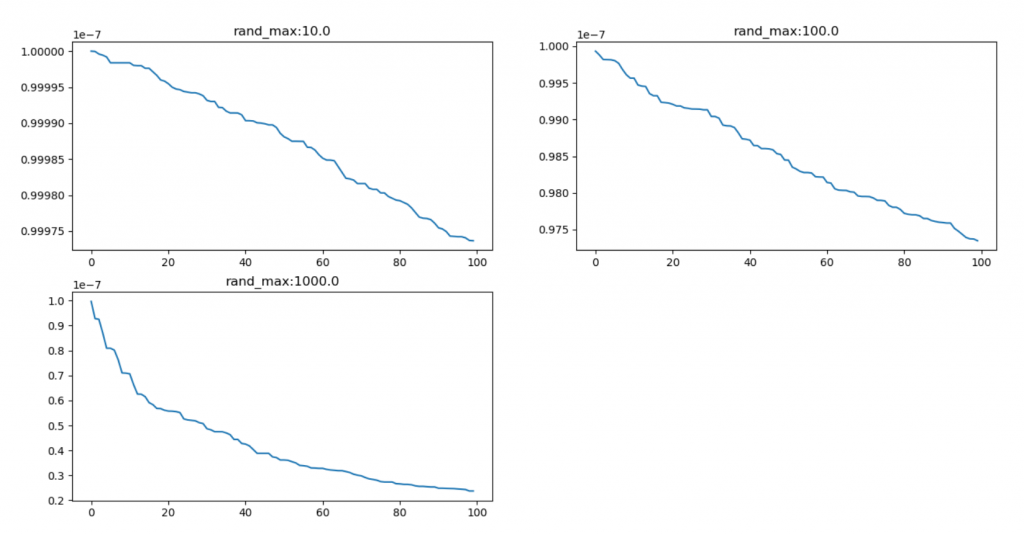``````lr = 1
stand = [
np.random.randint(0, 10, 100),
np.random.randint(0, 100, 100),
np.random.randint(0, 1000, 100)
]

for index in range(len(stand)):
pre = 0
pre_list = []
for step in range(100):
pre = pre + stand[index][step] * stand[index][step]
update_lr = lr / (pre + 1e7)
pre_list.append(update_lr)

plt.subplot(2, 2, index + 1)
plt.title("rand_max:" + str(np.math.pow(10, index + 1)))
plt.plot(np.arange(0, 100, 1), pre_list)

plt.show()
``````

### Tensorflow應用

``````def train(loss, index):
#return tf.train.MomentumOptimizer(learning_rate, momentum=0.9).minimize(loss, global_step=index)
``````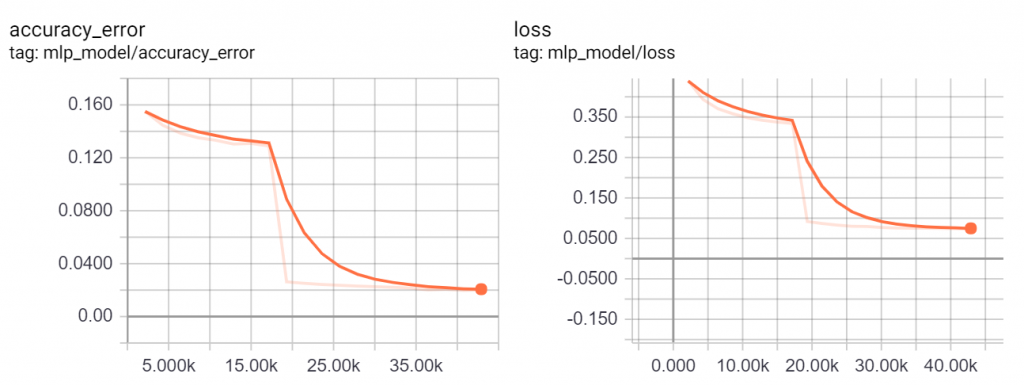## RMSProp

`RMSProp``AdaGrad`的改善，它加入了指數加權，也能說是`AdaGrad``Momentum`結合。它與`AdaGrad`差別在於將歷史梯度的影響變大，減少了訓練時的震盪(`Momentum`特性)。書中還提到了`Nesterow`這裡先略過。

### 公式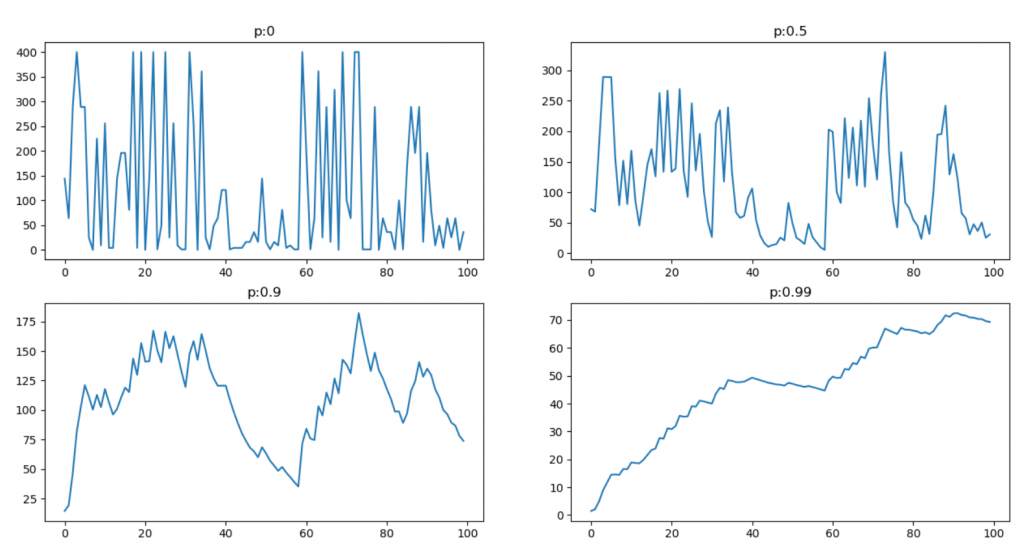``````lr = 1
p = [0, 0.5, 0.9, 0.99]
stand = np.random.randint(-20, 10, 100)

for index in range(len(p)):
pre = 0
pre_list = []
for step in range(100):
pre = p[index] * pre + (1 - p[index]) * stand[step] * stand[step]
pre_list.append(pre)

plt.subplot(2, 2, index + 1)
plt.title("p:" + str(p[index]))
plt.plot(np.arange(0, 100, 1), pre_list)

plt.show()
``````

### Tensorflow應用

``````def train(loss, index):
return tf.train.RMSPropOptimizer(learning_rate, decay=0.9).minimize(loss, global_step=index)
#return tf.train.MomentumOptimizer(learning_rate, momentum=0.9).minimize(loss, global_step=index)
``````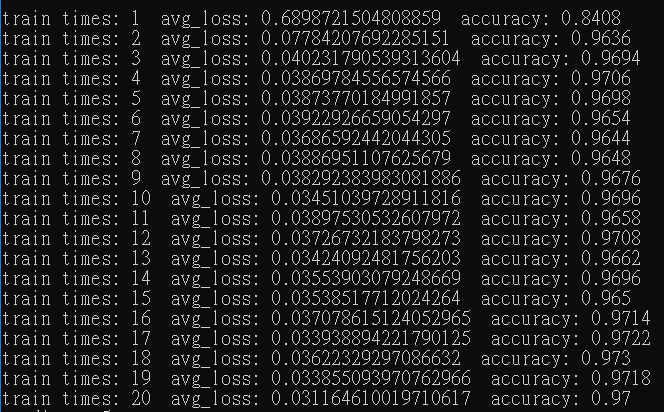### 推倒修正公式

使用`二階矩`來推倒。

1. 展開公式，`beta2`往前推導，第一項為`beta2^目前計算次數-1`，第二項為`beta2^目前計算次數-2`。每多計算一次前面的梯度`beta2`也會多乘上一次，所以展開才是此公式。
2. 使用sigma代替加總並提出係數(`1-beta2`)。• 觀點一：使用原先公式可知道v=beta2*舊資料 + (1-beta2)g^2組成，這裡直接假設v=Xg^2，直接帶入上述推導公式，可以將g中的k都取代為i。
• 觀點二：忽略歷史梯度，計算真實的`二階矩`，這時候帶入上述推導公式，可以將g中的k都取代為i，但beta2還是要留著(雖然我們假設0但留著不影響推倒)。

1. 將g提到前面。
2. 將sigma展開後，使用代數3. 最後除上代數即是修正後的`二階矩`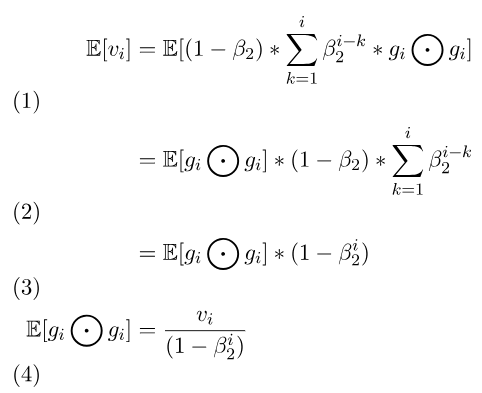### Tensorflow應用

`Adam`對於不穩定的數據和較複雜的網路有比較好的解，將MLP改為使用5層隱藏層每層200個神經元。若使用上述方法訓練則要調整學習率，且訓練速度並不快(無調整學習率，不到10%正確率)，但使用`Adam`在這裡則不需要調整，與其他相比收斂速度算快，如下圖訓練十次結果。

``````def train(loss, index):
return tf.train.AdamOptimizer(learning_rate, beta1=0.9, beta2=0.999, epsilon=1e-08).minimize(loss, global_step=index)
#return tf.train.RMSPropOptimizer(learning_rate, decay=0.9).minimize(loss, global_step=index)
#return tf.train.MomentumOptimizer(learning_rate, momentum=0.9).minimize(loss, global_step=index)
``````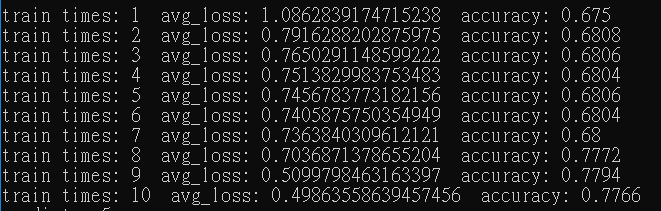# 參考網址與書籍

 https://www.tensorflow.org/api_docs/python/tf
 籃子軒(譯者)（2018）。Deep Learning深度學習基礎｜設計下一代人工智慧演算法。台灣：歐萊禮。
 https://upmath.me/